• 层次分析法 1.主要解决哪类问题？ 评价类 评价类问题要明确：评价目标是什么？为达到这个目标可选方案？评价准则或标准是什么？ 2.原理/思路是啥？ eg.小明高考完如何选择一个学校？/如何选择一个旅游目的地? ...
层次分析法
1.主要解决哪类问题？
评价类
评价类问题要明确：评价的目标是什么？为达到这个目标的可选方案？评价的准则或标准是什么？
2.原理/思路是啥？
eg.小明高考完如何选择一个学校？/如何选择一个旅游目的地?
可以思考到，并得到表格：定义指标+权重评分→依据得分进行选择
但是如何得到指标的权重？→两个两个指标进行比较，根据两两比较的结果来推算权重
如何两两比较，见图：有了判断矩阵，就可以计算出权重，如何计算出各个旅游地在景色方面所占的权重？如下图：在使用判断矩阵求权重前，先要进行一致性检验。什么是一致性？如何进行一致性检验？如何选择指标→依据题目的背景材料、常识、网络上搜集到的参考资料
权重的计算→算数平均数法、几何平均数法、特征值法（最常用）3.建模步骤是啥？
首先分析系统中各因素之间的关系，建立系统的递阶层次结构，如图：其次，对于同一层次的个元素之间两两比较，构造两两比较矩阵（判断矩阵）
最后进行一致性检验，得到权重，进行计算，做出决策/判断
4.论文中应该怎么写？
5.模型的优缺点？
优：可以进行无数据又需要比较的情况；定性问题之间进行量化（即用数字表示）比较；
缺：评价的决策层不可以太多；如果决策层中指标的数据是已知的，那么我们如何利用这些数据使评价更准确？如图：6.代码讲解？
7.模型拓展
eg.从九个因素中选取最重要的5个因素，就可以用层次分析法，选取权重最大的那几个。
层次分析法常是用于论文中的某一步，而不会全篇论文。
准则层和方案层之间可以有多重变化，如图：展开全文算法 数学建模
• 层次分析法 Analytic Hierarchy Process AHP 二 层次分析法的基本步骤 三 层次分析法建模举例 * * T.L.saaty 层次分析法建模 一 问题的提出 日常生活中有许多决策问题决策是指在面临多种 方案时需要依据一定的标准...
• 为了研究各因素对煤与瓦斯突出的影响程度,文章应用层次分析法的基本原理建立了煤与瓦斯突出指标的评价模型,并进行了实际应用,研究结果表明:采用该评价模型选择的评价指标能够很好地反映煤层突出的危险性,另外,可以...
• 基于区间数层次分析法的基本原理,全面综合考虑了煤矿安全生产的各种因素,应用区间数层次分析,将各指标两两比较得出区间数判断矩阵;将判断矩阵的特征向量作为煤矿安全评价因子的权重范围,构建了煤矿安全评价指标体系....
• 从系统工程的角度出发,在应用层次分析法的基础上构建了矿井通风系统安全评价模型,确定了矿井通风系统安全评价指标体系和指标的权重。结果表明:通风网络阻力分布安全性是影响矿井通风系统安全的首要因素,其次是风量...
• 熵值法是一种依据各指标值所包含信息量大小，来确定决策指标权重客观赋权法。 灰色系统理论研究对象是部分信息已知而部分信息未知“贫信息”’不确定性系统，利用已知信息来确定系统未知信息...层次分析法
熵值法
在信息论中，熵值能够很好的反映信息无序化程度，其值越小，系统无序度越低，信息的效用值越大，其权重也相应越大；反之，其值越大，系统无序度越高，信息的效用值越小，权重也越小。
步骤
熵值法赋权计算步骤如下：
1）确定$m$个需进行评价的样本对象和$n$个评价指标，构建一个对应的判断矩阵$R=(x_{ij})_{mn},(i=1,2,...,m，j=1,2,...n)$.
2）将判断矩阵进行标准化，即将各指标进行同度量化。该模型采用比重法对指标判断矩阵原始值进行标准化：
$P_{i j}=\frac{x_{i j}}{\sum_{i=1}^{m} x_{i j}}$
3）计算第$j$项指标的熵值，利用熵值公式计算评价指标的熵值：
$e_{j}=-k \sum_{i=1}^{m} P_{i j} \ln P_{i j}, \text { 其中 } k=\frac{1}{\ln m}(i=1, \ldots, m ; j=1, \ldots, n)$
4）计算第$j$项指标的差异性系数$g_j$。熵值越小，指标间差异性越大，指标在综合评价中所起作用就越大：
$g_j=1-e_j$
5）用熵值法估算各指标的权重，本质上是计算各项指标的价值系数，最后可以得到第$j$项指标的权重系数$\omega_j$：
$\omega_{j}=\frac{g_{j}}{\sum_{i=1}^{n} g_{j}}$
python实现
def Entropy_(standardized_data,weight_num=None,up_layer_num=None,weight_interval=None):
'''
:param standardized_data: 需要计算权重的数据
:param weight_num: 权重个数
:param up_layer_num: 是否要计算第二层权重，需要则传入上层指标个数
:param weight_interval: 若要计算第二层权重，传入第一层指标个数区间
:return: 第一层的差异性系数g，权重w,熵值e
'''
rows, cols = standardized_data.shape
k = 1/math.log(rows)
standardized_data_arr = np.array(standardized_data)
standardized_data_log = np.log(standardized_data_arr)
use_data = pd.DataFrame(standardized_data_arr*standardized_data_log)
use_data.loc['col_sum'] = use_data.apply(lambda x:x.sum())
#计算熵值
e = use_data.loc['col_sum']*(-k)
#计算差异性系数
g = 1-e
if up_layer_num is not None:
#权重计算
g_arr = np.zeros(shape=[up_layer_num])
for i in range(up_layer_num):
for j in weight_interval[i]:
g_arr[i] = g_arr[i] + g.iloc[j]
#权重
w = np.zeros(weight_num)
for i in range(up_layer_num):
for j in weight_interval[i]:
w[j] = g[j] / g_arr[i]
else:
# 权重计算
w = np.array([i/np.sum(g) for i  in g])
w = pd.DataFrame(w)
return g,e,w

灰色关联分析
灰色系统理论的研究对象是部分信息已知而部分信息未知的“贫信息”’不确定性系统，利用已知信息来确定系统的未知信息，对样本量没有严格的规定，也不要求服从任何典型分布规律，且不会出现量化结果与定性分析不一致的情况。而关联分析是灰色系统分析、评级和决策的基础，其基本思想是根据数据指标呈现出的序列曲线的几何形状相似程度来判断两个指标之间的联系是否紧密，通过关联度表征两个事物间的关联程度。
灰色关联度分析步骤
1）延用熵值法中构建的原始数据判断矩阵$R=(x_{ij})_{m×n}$,矩阵如下：
$R=\left[\begin{array}{ccc} x_{11} & \cdots & x_{1 n} \\ \vdots & \ddots & \vdots \\ x_{m 1} & \cdots & x_{m n} \end{array}\right]$
2）选取最优指标集
各评价指标的最优值组成的集合称为最优集，它是评价对象比较的基准，记录为
$F=k_j,(j=1,2,3,...,n)$
式子中$k_j$为第$j$个指标的最优值。在最优值的选取过程中，若指标为成本型指标，则最优值选取当中的最小值；若指标为效益型指标，则最优值选取当中的最大值。最优指标集和各待评价对象的指标组成一个矩阵 D：
$D=\left[\begin{array}{ccc} k_{1} & \cdots & k_{n} \\ x_{1} & \cdots & x_{n} \\ \vdots & \ddots & \vdots \\ x_{m 1} & \cdots & x_{m n} \end{array}\right]$
式中，$x_{ij}$为第$i$个评价对象的第$j$个指标的原始数值.
3）计算关联系数，求取评判矩阵
根据灰色系统理论，将最优数列作为参考数列，将其他数列作为被比较数列，用关联分析法分别求得第$i$个评价对象的第$j$个指标与第$j$个最优指标的关联系数$e_{ij}$，计算公式如下：
$e_{i j}=\frac{\min _{i} \min _{j}\left|k_{j}-x_{i j}\right|+\rho \max _{i} \max _{j}\left|k_{j}-x_{i j}\right|}{\left|k_{j}-x_{i j}\right|+\rho \max _{i} \max _{j}\left|k_{j}-x_{i j}\right|}$
式中，$\rho$为分辨系数，其取值范围在[0,1]，一般取$\rho=0.5$；$\min _{i} \min _{j}\left|k_{j}-x_{i j}\right|$为两级最小差，$\max _{i} \max _{j}\left|k_{j}-x_{i j}\right|$为两级最大差。
则各指标的评判矩阵即关联系数记录为$E$，如下：
$E=\left[\begin{array}{ccc} e_{11} & \cdots & e_{1 n} \\ \vdots & \ddots & \vdots \\ e_{m 1} & \cdots & e_{m n} \end{array}\right]$
式中，$e_{ij}$为第$i$个样本第$j$个指标与第$j$个最优指标的关联系数。
4）建立灰色单层次评判矩阵
$RX=E×W$
式中，$R X=\left[r_{1}, r_{2}, \ldots, r_{m}\right]^{T}$为$m$个被评价对象的综合评价结果向量；即$r_i = \sum_{i=1}^{m} \omega_{j}e_{ij}$；$W=\left[\omega_1,\ldots,\omega_n \right]$为$n$个评价指标的权重分配向量，其中$\sum_{j=1}^{n} \omega_{j}=1$。
5）根据实际指标情况，可建立多层评判标准
当评价对象的各个指标间分为不同层次时，需要采用多层次综合评价法。灰色多层次综合评判是以单层次综合评价为基础，需将单层次评价结果矩阵作为下一个层次的原始指标列，再重复进行下一层次单层评判计算，依次类推至最高层。若灰色关联度越大，说明该评价对象与参考指标越接近，即第$i$个评价对象越优于其他，通过关联度这指标排出样本之间的优劣次序。
python实现
暂无

层次分析法
层次分析法，简称AHP，是指将与决策总是有关的元素分解成目标、准则、方案等层次，在此基础之上进行定性和定量分析的决策方法。
层次分析法属于主观赋权法，具有简洁、系统性分析的优点，但主观性强，不易令人信服，并且当数据量较大时，权重难以确定。
计算步骤
1）建立层次结构模型
将决策的目标、考虑的因素（决策准则）和决策对象按它们之间的相互关系分为最高层、中间层和最低层，绘出层次结构图。 最高层是指决策的目的、要解决的问题。 最低层是指决策时的备选方案。 中间层是指考虑的因素、决策的准则。对于相邻的两层，称高层为目标层，低层为因素层。
2）构造判断(成对比较)矩阵
两两因素相互比较，对此时采用相对尺度，以尽可能减少性质不同的诸因素相互比较的困难，以提高准确度。如对某一准则，对其下的各方案进行两两对比，并按其重要性程度评定等级。 为要素与要素重要性比较结果，表1列出Saaty给出的9个重要性等级及其赋值。按两两比较结果构成的矩阵称作判断矩阵：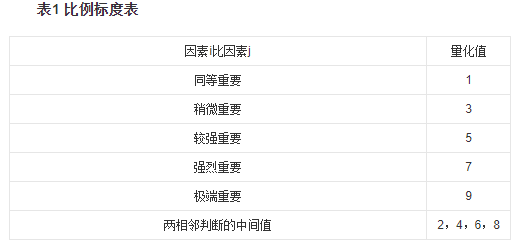3）层次单排序及其一致性检验
对应于判断矩阵最大特征根$\lambda_{max}$的特征向量，经归一化后记为$W$。$W$的元素为同一层次因素对于上一层次因素某因素相对重要性的排序权值，这一过程称为层次单排序。能否确认层次单排序，则需要进行一致性检验，所谓一致性检验是指对$A$确定不一致的允许范围。其中，$n$阶一致阵的唯一非零特征根为$n$；$n $阶正互反阵$A$的最大特征根 $\lambda ≥n$， 当且仅当$\lambda = n$时，$A$为一致矩阵。
一致性指标用CI计算，CI越小，说明一致性越大，计算公式为：
$CI= \frac{\lambda-n}{n-1}$
$CI=0$，有完全的一致性；$CI$接近于$0$，有满意的一致性；$CI$ 越大，不一致越严重。
为衡量$CI$ 的大小，引入随机一致性指标 $RI$：
$RI=\frac{CI_1+CI_2+\dots+CI_n}{n}$
其与矩阵阶数的关系，一般情况下，矩阵阶数越大，则出现一致性随机偏离的可能性也越大，其对应关系如表2：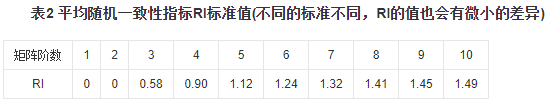考虑到一致性的偏离可能是由于随机原因造成的，因此在检验判断矩阵是否具有满意的一致性时，还需将$CI$和随机一致性指标$RI$进行比较，得出检验系数$CR$，公式如下：
$CR=\frac{CI}{RI}$
一般，如果$CR<0.1$ ，则认为该判断矩阵通过一致性检验，否则就不具有满意一致性。
4）层次总排序及其一致性检验
python实现
# python3.7
# -*- coding: utf-8 -*-
#@Author    : huinono
#@Software  : PyCharm

import warnings
import numpy as np
import pandas as pd
import matplotlib as mpl
import matplotlib.pyplot as plt

warnings.filterwarnings('ignore')

mpl.rcParams['font.sans-serif'] = 'SimHei'
mpl.rcParams['axes.unicode_minus'] = 'False'

plt.rcParams['font.sans-serif'] = 'SimHei'
plt.rcParams['axes.unicode_minus'] = 'False'

'''
1、构建判断矩阵：准则层和方案层判断矩阵
2、对方案层判断矩阵求特征向量
3、计算最大特征值与层次单排序的一致性检验
4、层次总排序的一致性检验
5、求得权重
'''
class AHP(object):
def __init__(self):
self.RI = [0,0,0.58,0.90,1.12,1.24,1.32,1.41,1.45]

def VectorsCal(dself,data):
data_sum = np.sum(data,axis=1)
data_mean = data_sum/data.shape
data_vecs = data_mean/np.sum(data_mean)
return data_vecs

def SOConsistencyCheck(self,vals_max,n):
CI = (vals_max-n)/(n-1)
if CI == 0:
CR=0
RI = self.RI[n - 1]
else:
RI = self.RI[n-1]
CR = CI/RI
return CR,CI,RI

def weightCal(self,weight_interval,weight_num,JudgmentMatrix_all):
'求特征向量'
matrix_vecs_ = [self.VectorsCal(con) for con in JudgmentMatrix_all]
'计算最大特征值和单排序一致性检验'
matrix_vals_vecs = [np.linalg.eig(con) for con in JudgmentMatrix_all]
matrix_vals_max = [np.max(vals_vecs) for vals_vecs in matrix_vals_vecs]
matrix_CR = [self.SOConsistencyCheck(matrix_vals_max[i], JudgmentMatrix_all[i].shape) for i in range(len(matrix_vals_max))]
matrix_CI = [self.SOConsistencyCheck(matrix_vals_max[i], JudgmentMatrix_all[i].shape) for i in range(len(matrix_vals_max))]
matrix_RI = [self.SOConsistencyCheck(matrix_vals_max[i], JudgmentMatrix_all[i].shape) for i in range(len(matrix_vals_max))]
'总排序一致性检验'
C1 = matrix_vecs_[-1].reshape(-1, 1)
matrix_zero = np.zeros(weight_num)
P = []
length = len(weight_interval)
for i in range(length):
if i == 0:
P1 = np.concatenate([matrix_vecs_[i], matrix_zero[weight_interval[i][-1] + 1:]], axis=0).reshape(-1, 1)
P.append(P1)
elif i == length-1:
P3 = np.concatenate([matrix_zero[:weight_interval[i]], matrix_vecs_[i]], axis=0).reshape(-1, 1)
P.append(P3)
else:
P2 = np.concatenate([matrix_zero[:weight_interval[i]], matrix_vecs_[i], matrix_zero[weight_interval[i][-1] + 1:]],axis=0).reshape(-1, 1)
P.append(P2)
weight_one = np.dot(np.concatenate(P, axis=1), C1)
if np.dot(matrix_CI[1:], C1) == 0 and np.dot(matrix_RI[1:],C1) == 0:
CR1 = 0
else:
CR1 = np.dot(matrix_CI[1:], C1) / np.dot(matrix_RI[1:],C1)
return weight_one,matrix_CR,CR1,matrix_vecs_,matrix_vals_max



展开全文python 算法
• 文章将模糊综合评价法与层次分析法相结合,建立液压支架安全评价模型,采用定性与定量相结合的层次分析法确定了指标体系中各因素权重,对在役液压支架安全性进行评价。通过实例液压支架分析,证明其评价结果和实际...
• 层次分析法的基本思路4.层次分析法的基本步骤4.1 建立层次结构模型4.2 构建成对比较矩阵4.3 做一致性检验 1.问题提出 日常生活中有很多的决策问题。决策是指在面临多种方案时需要依据一定的标准（一个或多个）选择某...
快速索引烂1.问题提出2.概念提出3.层次分析法的基本思路4.层次分析法的基本步骤4.1 建立层次结构模型4.2 构建成对比较矩阵4.3 做一致性检验4.4 层次总排序及决策5.参考文献

1.问题提出
日常生活中有很多的决策问题。决策是指在面临多种方案时需要依据一定的标准（一个或多个）选择某一种方案。
例，择业：
面临毕业，可能有高校、科研单位、企业等单位可以去选择，一般依据工作环境、工资待遇、发展前途等因素择业。
2.概念提出
3.层次分析法的基本思路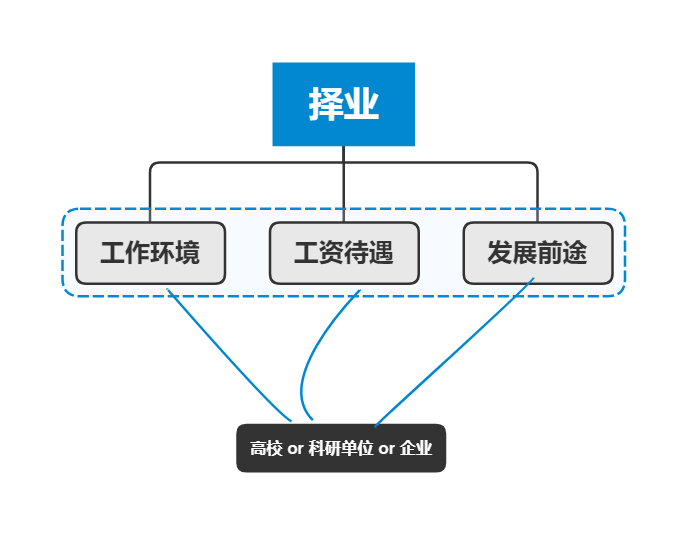4.层次分析法的基本步骤
4.1 建立层次结构模型
将问题包含的因素分层：
1.最高层（解决问题的目的）；
2.中间层（选择为实现总目标而采取的各种措施、方案所必须遵循的准则。也可称策略层、约束层、准则层等）；
3.最低层（用于解决问题的各种措施、方案等）。
如上例：
假设小明选择了高校、科研单位、企业作为择业目标，以工作环境，工作待遇，发展前途三个指标.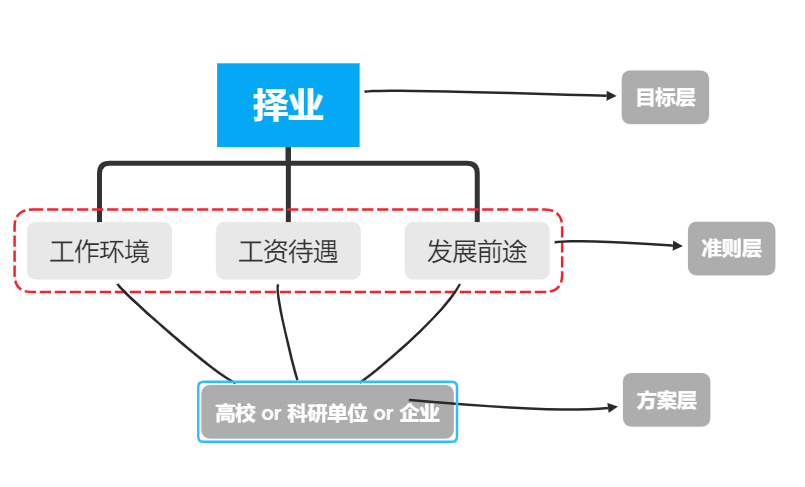4.2 构建成对比较矩阵
在确定各层次各因素之间的权重时，如果只是定性的结果（个人理解是直接判断每个因素的占比），则常常不容易被别人接受，因而Saaty等人提出一致矩阵法。
1.不把所有因素放在一起比较，而是两两相互比较。
2.对此时采用相对尺度，以尽可能减少性质不同的诸因素相互比较的困难，以提高准确度。
引入判断的标度方法：

评估尺度
定义
说明

1
同等重要
两要素的贡献程度具同等重要性

3
稍微重要
经验与判断稍微偏好某一要素

5
颇为重要
经验与判断强烈偏好某一要素

7
极为重要
实际显示非常强烈偏好某一要素

9
绝对重要
有足够证据肯定绝对偏好某一要素

2,4,6,8
相邻尺度之中间值
介于两种判断之间

工作环境
工资待遇
发展前途

工作环境
1

工资待遇

1

发展前途

1

再次引用上例，如果我们觉得：
1.工资待遇比工资环境稍微重要，工资待遇/工资环境=3
2.发展前途和工资待遇介于稍微重要和同等重要之间，发展前途/工资待遇=2
3.发展前途比工资环境极为重要，发展前途/工资待遇=7
得到下表：

工作环境
工资待遇
发展前途

工作环境
1
1/3
1/7

工资待遇
3
1
1/2

发展前途
7
2
1

得到矩阵：记为$A$， 对应的元素为$a_{ij}$
$A = \left[ \begin{matrix} 1&1/3&1/7\\ 3&1&1/2 \\ 7&2&1 \end{matrix} \right]$
其中： $a_{ij}$表示，与指标$j$相比，$i$的重要程度。
4.3 做一致性检验
从理论上分析得到：如果$A$是完全一致的成对比较矩阵，应该有
$a_{12}a_{23}=a_{13}$
$1/2 *1/3=1/6$
而实际上，
$a_{13} = 1/7$
例如：
工资待遇/工资环境=3
发展前途/工资待遇=2
此时，如果完全一致，发展前途/工资待遇 应该等于6，而我们标度，发展前途/工资待遇=7

对比较矩阵时要求满足完全满足是不太可能的。因此退而要求成对比较矩阵有一定的一致性，即可以允许成对比较矩阵存在一定程度的不一致性。
检验步骤：
1.计算衡量一个成对比较矩阵 A （n>1 阶方阵）不一致程度的指标CI
$CI=\frac{\lambda_{max}(A)-n}{n-1}$
2.查找对应的平均随机一致性指标RI

n
1
2
3
4
5
6
7
8
9
10
11
12
13
14
15

RI
0
0
0.52
0.89
1.12
1.26
1.36
1.41
1.46
1.49
1.52
1.54
1.56
1.58
1.59

3.计算一致性比例CR
$CR=\frac{CI}{RI}$
判断方法如下：
当CR<0.1时，判定成对比较阵 A 具有满意的一致性，或其不一致程度是可以接受的；否则就调整成对比较矩阵 A，直到达到满意的一致性为止。
对上例矩阵
$A = \left[ \begin{matrix} 1&1/3&1/7\\ 3&1&1/2 \\ 7&2&1 \end{matrix} \right]$
计算得到$\lambda_{max}(A)=3.003,CI=\frac{\lambda_{max}(A)-3}{3-1}=0.0013$,查得$RI=0.52$，
$CR=\frac{CI}{RI}=\frac{0.018}{1.12}=0.0025<0.1$
这说明 A 不是一致阵，但 A 具有满意的一致性，A 的不一致程度是可接受的。
4.4 层次总排序及决策
现在完整解决例题，从高校和企业选择一个最符合上述3个因素的就业地点。
对此，对高校和企业分别比较它们的工作环境，工资待遇，发展前途.

工作环境
高校
科研单位
企业

高校
1
3
5

科研单位
1/3
1
4

企业
1/5
1/4
1

得到方阵：
$B1 = \left[ \begin{matrix} 1&3&5\\ 1/3&1&4\\ 1/5&1/4&1 \end{matrix} \right]$

工资待遇
高校
科研单位
企业

高校
1
1/7
1/5

科研单位
7
1
3

企业
5
1/3
1

得到方阵：
$B2 = \left[ \begin{matrix} 1&1/7&1/5\\ 7&1&3\\ 5&3&1 \end{matrix} \right]$

发展前途
高校
科研单位
企业

高校
1
1/5
1/3

科研单位
5
1
2

企业
3
1/2
1

得到方阵：
$B3 = \left[ \begin{matrix} 1&1/5&1/3\\ 5&1&2\\ 3&1/2&1 \end{matrix} \right]$

同时也计算这三个判断矩阵的$CR$,经计算
$CR(B1) = 0.08246797115384596$
$CR(B2)= 0.09039914423076918$
$CR(B3)=0.003552500000000206$
都可以接受
计算权重：
1.将判断矩阵按照列归一化
(每一个元素出意其所在列的和)

工作环境
高校
科研单位
企业

高校
0.652
0.706
0.5

科研单位
0.217
0.235
0.4

企业
0.130
0.059
0.1

2.将同属于一个因素的值相加并除以$n$得

工作环境
权重

高校
0.610

科研单位
0.284

企业
0.096

同理，

工资待遇
权重

高校
0.072

科研单位
0.589

企业
0.339

发展前途
权重

高校
0.109

科研单位
0.570

企业
0.321

指标权重

工作环境
0.092

工资待遇
0.282

发展前途
0.626

汇总结果，得到权重矩阵

指标权重
高校
科研单位
企业

工作环境
0.092
0.610
0.284
0.096

工资待遇
0.282
0.072
0.589
0.339

发展前途
0.626
0.109
0.570
0.321

计算得，高校=0.092x0.060+0.282x0.072+0.626x0.109=0.145
同理，科研单位=0.549
企业=0.305
综上，择业应该选择科研单位
5.参考文献

层次分析法-MBA智库 https://wiki.mbalib.com/wiki/AHP
数学建模清风课程笔记


展开全文数学模型 数学建模
• 层次分析法属于运筹学理论，简称为AHP，其主要思路是将决策总有关元素分解为多个层次，基本的AHP主要分为三层目标层，准则层，方案层。在此基础上进行定量与定性分析。最终得出决策依据。该方法是美国运筹学家匹茨...
简介
层次分析法属于运筹学理论，简称为AHP，其主要思路是将决策总有关的元素分解为多个层次，基本的AHP主要分为三层目标层，准则层，方案层。在此基础上进行定量与定性分析。最终得出决策依据。该方法是美国运筹学家匹茨堡大学教授萨蒂于20世纪70年代初，在为美国国防部研究“根据各个工业部门对国家福利的贡献大小而进行电力分配”课题时，应用网络系统理论和多目标综合评价方法，提出的一种层次权重决策分析方法。
（最简单的应用是boy们评价美女，girl们评价帅哥哦~）
内容介绍层次分析法是指将一个复杂的多目标决策问题作为一个系统，将目标分解为多个目标或准则，进而分解为多指标（或准则、约束）的若干层次，通过定性指标模糊量化方法算出层次单排序（权数）和总排序，以作为目标（多指标）、多方案优化决策的系统方法。
层次分析法是将决策问题按总目标、各层子目标、评价准则直至具体的备投方案的顺序分解为不同的层次结构，然后用求解判断矩阵特征向量的办法，求得每一层次的各元素对上一层次某元素的优先权重，最后再加权和的方法递阶归并各备择方案对总目标的最终权重，此最终权重最大者即为最优方案。
层次分析法比较适合于具有分层交错评价指标的目标系统，而且目标值又难于定量描述的决策问题。
基本原理
AHP主要根据问题的性质和要达到的总目标，将问题划分为不同组成因素（简单点说就是小学学的课文，把大问题划分为小问题，然后逐一解决，意思差不多哈~）。并按照因素间的相互关联影响以及隶属关系将因素按不同层次聚集组合，形成一个多层次的分析结构模型，从而最终使问题归结为最低层(供决策的方案、措施等)相对于最高层(总目标)的相对重要权值的确定或相对优劣次序的排定。
具体步骤
1.建立层次结构模型
将决策的目标、考虑的因素（决策准则）和决策对象按它们之间的相互关系分为最高层、中间层和最低层，绘出层次结构图。最高层是指决策的目的、要解决的问题。最低层是指决策时的备选方案。中间层是指考虑的因素、决策的准则。对于相邻的两层，称高层为目标层，低层为因素层。
2.构造判断(成对比较)矩阵
在确定各层次各因素之间的权重时，如果只是定性的结果，则常常不容易被别人接受，因而Saaty等人提出一致矩阵法，即不把所有因素放在一起比较，而是两两相互比较，对此时采用相对尺度，以尽可能减少性质不同的诸因素相互比较的困难，以提高准确度。如对某一准则，对其下的各方案进行两两对比，并按其重要性程度评定等级。为要素i与要素j重要性比较结果（即量化值比较结果）=i的量化值/j的量化值重要性比较结果，表1列出Saaty给出的9个重要性等级及其赋值。按两两比较结果构成的矩阵称作判断矩阵。判断矩阵具有如下性质：
判断矩阵元素的标度方法如下：
表1 比例标度表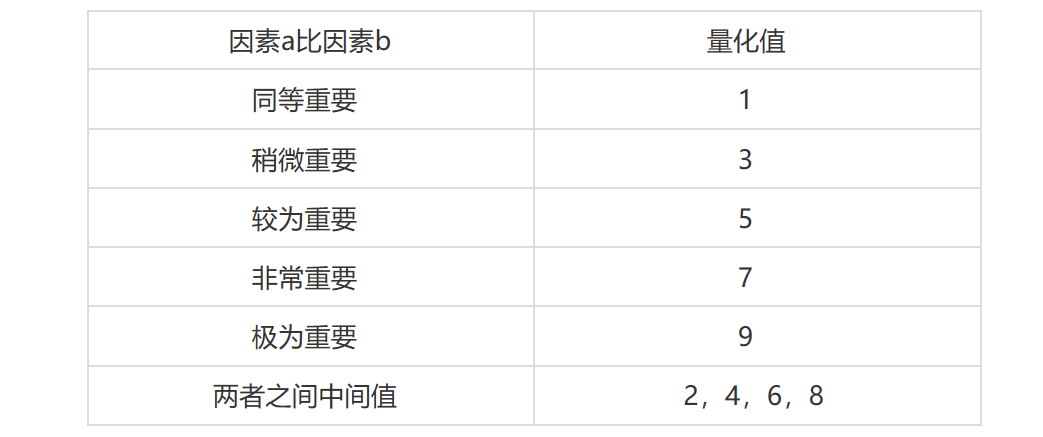层次单排序及其一致性检验
对应于判断矩阵最大特征根的特征向量λmax，经归一化(使向量中各元素之和等于1)后记为W。W的元素为同一层次因素对于上一层次因素某因素相对重要性的排序权值，这一过程称为层次单排序。能否确认层次单排序，则需要进行一致性检验，所谓一致性检验是指对A确定不一致的允许范围。其中，n阶一致阵的唯一非零特征根为n；n 阶正互反阵A的最大特征根， 当且仅当时，A为一致矩阵。
由于λ连续的依赖于aij，
则λ 比n 大的越多，A的不一致性越严重，一致性指标用CI计算，CI越小，说明一致性越大。用最大特征值对应的特征向量作为被比较因素对上层某因素影响程度的权向量，其不一致程度越大，引起的判断误差越大。因而可以用 λ-n 数值的大小来衡量A 的不一致程度。定义一致性指标为：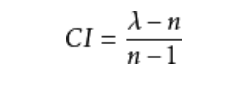CI=0，有完全的一致性；CI 接近于0，有满意的一致性；CI 越大，不一致越严重。
为衡量CI 的大小，引入随机一致性指标 RI：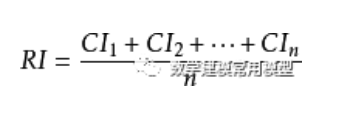其中，随机一致性指标RI和判断矩阵的阶数有关，一般情况下，矩阵阶数越大，则出现一致性随机偏离的可能性也越大，其对应关系如表2：
表2 平均随机一致性指标RI标准值(不同的标准不同，RI的值也会有微小的差异)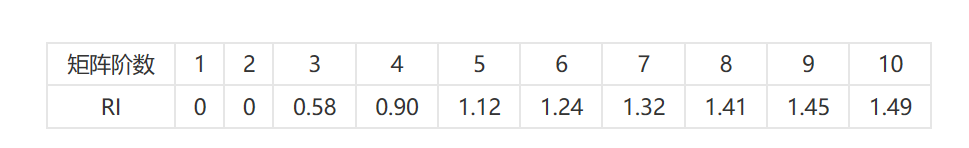考虑到一致性的偏离可能是由于随机原因造成的，因此在检验判断矩阵是否具有满意的一致性时，还需将CI和随机一致性指标RI进行比较，得出检验系数CR，公式如下：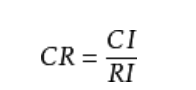一般，如果CR<0.1 ，则认为该判断矩阵通过一致性检验，否则就不具有满意一致性。
4.层次总排序及其一致性检验
计算某一层次所有因素对于最高层(总目标)相对重要性的权值，称为层次总排序。这一过程是从最高层次到最低层次依次进行的。这些东东在百度百科都有详细的介绍，来源，起源啥的，我就不多赘述了，大家看我写的例题就好啦。看一遍基本就懂啦。
—— —— —— —— —— —— —— —— —— —— —— —— —— —介绍就这么多我们拿具体的例题来讲具体计算步骤以最简单的例子，假设我们要买一根钢笔首先我们明确   比例标度表
选择钢笔
质量a1   颜色a2     价格a3  品牌a4   外形a5    实用a6
钢笔1  钢笔2  钢笔3  钢笔4
构建出模型
买钢笔                                                    目标层
质量a1   颜色a2   价格a3   品牌a4    外形a5  实用度a6      指标层
钢笔1  钢笔2  钢笔3  钢笔4                          决策层    然后构建对比矩阵因为指标层拥有6个因素，所以我们设X={x1,x2,A,x6}要比较它们对上层某一准则（或目标）的影响程度，按照比例标度表确定它的量化值，例如我认为本题中质量非常重要所以我取质量的量化值为7即a1=7,因此同理，a2=5,a3=6,a4=7,a5=8,a6=4我们得出量化值即可构建对比矩阵为要素i与要素j重要性比较结果（即量化值比较结果）=i的量化值/j的量化值。例如a12=7/5。
判断矩阵具有如下性质：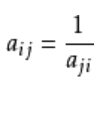得出矩阵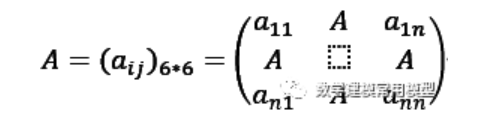我们得出此题目中指标层的判断矩阵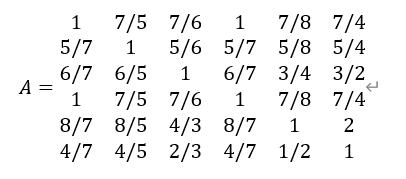我们进而求此矩阵特征值（此特征值就可做为权重）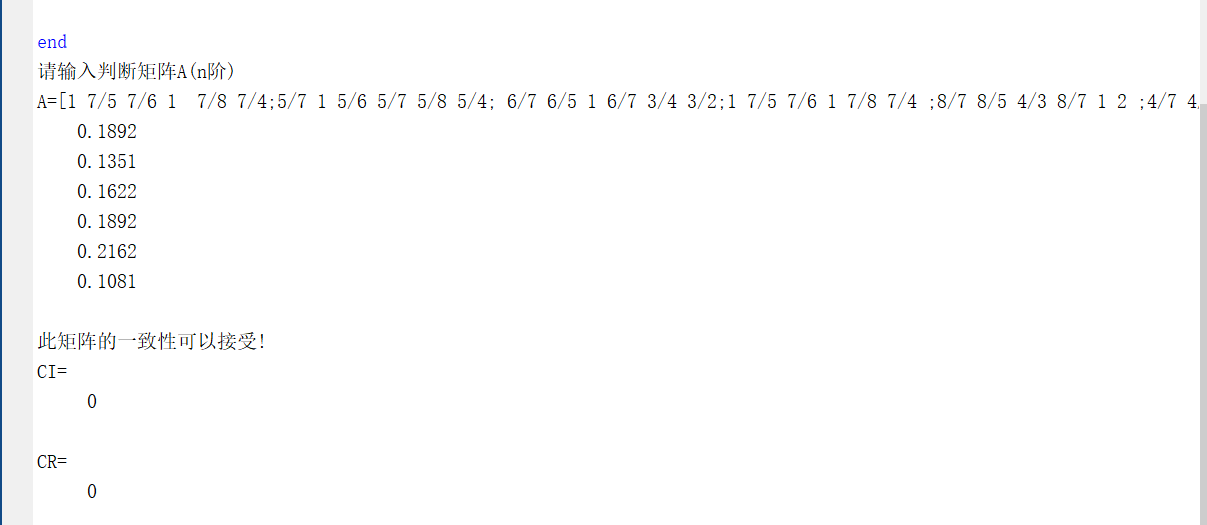由代码运算我们得出各指标权重而且通过一致性检验质量=0.1892颜色=0.1351价格=0.1622品牌=0.1892外形=0.2162实用度=0.1081接下来我们就构建我们的评价体系（这可以说是AHP最大的亮点之出，AHP经常被吐槽说过于简单，但是AHP在评价模型中可谓可操作性最大的模型，它最吸引人的地方就是评价模型，完全由建模者自己建立，这是AHP最大的亮点）此例题十分简单我们就简单以百分制来进行打分即可（如果大家对复杂完整的评价模型感兴趣的话可以关注我滴公众号，里面用到建模比赛的题目就是用AHP来解决的）
构建评价标准质量一级：80-100 质量二级：60-80 质量三级：40-60 质量四级：20-40其它标准以此建立（这只是最简的评价标准，大家在比赛或者实际中运用的要更加复杂，评价标准更加完善）
我们以钢笔一为例子假设质量：85  颜色：40 价格：100 品牌：20 外形：60 使用度70我们用分数乘上对应权重得出最终的综合评分XX=0.189285 +0.135140+0.1622100+0.189220+0.216260+0.108170=62.029我们对比四支钢笔的综合评分就可得出我们心目中最为心仪的一支笔。模型总结我们可以清楚的认识到AHP夹杂了大量的主观判断，大多数同学并不认可AHP就是因为主观判断其实并不严谨，所以我们改进了此模型，就像灰色-马尔可夫链式模型，运用组合模型可以大大增加模型严谨性。
在我们在构建对比矩阵时，我们需要对指标进行两两对比最终赋权，在此时我们可以采用德尔菲法，也称专家调查法，来进行赋权，运用此方法可以弥补AHP的客观缺陷。但是也许我们在比赛的时候并没有时间去采用此方法，但是我们完全可以根据已有文献来确定指标的对比赋权。这样AHP法的缺陷可以被大大的弥补。
也许，许多朋友说AHP很low，什么用AHP全部GG的。但是2017年美赛A题O奖论文，在某座非洲大坝年久失修可能坍塌的情况下，让你在1.修缮原有大坝2.拆掉大坝建一个新的3.拆掉大坝建一组新的小大坝来取代原有的一个大坝，中选择一个最优的。此论文就是运用AHP解决的，给大家它的Team number 55069，大家可以去看一下。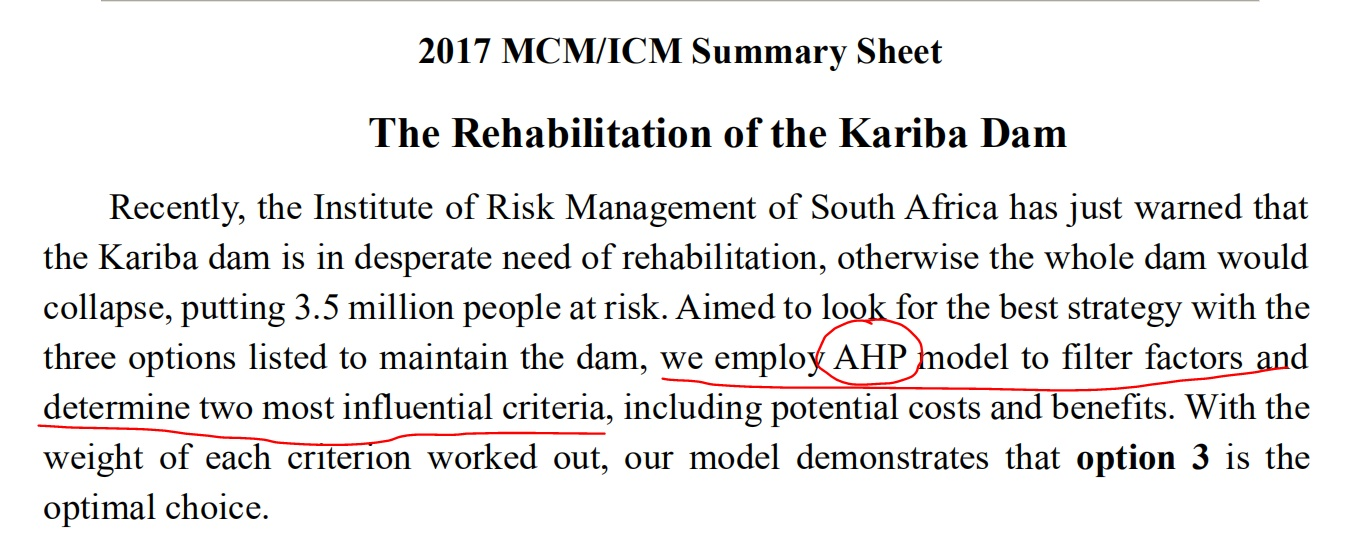同时2016年MCM C题Team Number50193，2014年B题O奖 Team Number26160，都是运用的AHP方法，我个人认为AHP最大亮点在于它的可操作性，不像线性回归，灰色预测等模型那样完全定式化，只要你构建的评价系统足够权威有理有据，而且十分巧妙，AHP根本不比神经网络，YOLO模型，这种高大上的模型差多少。
大家有需要可以看一下这个公众号，里面有基本数学建模模型，对大家可能有一定帮助，而且里面的代码经过验证都是可行的。实现代码（一位大神的代码）
disp('请输入判断矩阵A（n阶）');
A=input('A=');
[n,n]=size(A);
x=ones(n,100);
y=ones(n,100);
m=zeros(1,100);
m(1)=max(x(:,1));
y(:,1)=x(:,1);
x(:,2)=A*y(:,1);
m(2)=max(x(:,2));
y(:,2)=x(:,2)/m(2)
;p=0.0001;i=2;k=abs(m(2)-m(1));
while  k>p
i=i+1;
x(:,i)=A*y(:,i-1);
m(i)=max(x(:,i));  y(:,i)=x(:,i)/m(i);
k=abs(m(i)-m(i-1));
end
a=sum(y(:,i));
w=y(:,i)/a;
t=m(i);disp(w);
%以下是一致性检验
CI=(t-n)/(n-1);RI=[0 0 0.52 0.89 1.12 1.26 1.36 1.41 1.46 1.49 1.52 1.54 1.56 1.58 1.59];
CR=CI/RI(n);if CR<0.10
disp('此矩阵的一致性可以接受!');
disp('CI=');disp(CI);
disp('CR=');disp(CR);
end
————————————————
版权声明：本文为CSDN博主「一颗修行千年的葱」的原创文章，遵循 CC 4.0 BY-SA 版权协议，转载请附上原文出处链接及本声明。
原文链接：https://blog.csdn.net/weixin_40725491/article/details/82082466



展开全文算法
• 1.建立层次分析结构模型。常见有目标层-准则层-方案层模型。 2.构造成对比较矩阵。常用有1-9尺度。尺度大小取决于下层元素个数。 3.计算权向量并作一致性检验。引入一致性指标CI，CI越大，不一致越严重。引入...算法 数学建模 线性代数
• AHP基本思路AHP通常用于决策判断重要依据，在某些方面具有很好指导作用，尤其是定性判断指标转化为定量数据时。比如我们打算新建一座商场，初步认定了A、B、C三地，这三个地区各项指标都非常接近，此时决策者...
• 在明确模糊评价的基本理论的基础上,给出了以三角模糊数代替传统的评判标度的因素权重计算方法,结合桥梁状态评价需求提出了模糊数层次分析法综合评价桥梁状态流程及实施步骤。以所提出的方法对一钢筋混凝土桥梁结构...
• 然后，依据云计算安全需求和国家基本要求建立基于层次分析法的模糊综合评价模型，对某云服务平台进行安全性评估，得出该服务平台安全级别为“好”。最后，综合云计算环境的安全需求以及模型评价结果，针对云计算环境...安全需求 模糊综合评价
• 针对目前回采工作面预防与治理煤壁片帮工作的滞后性、盲目性等特点,建立回采工作面煤壁片帮预测体系,通过应用层次分析法和模糊数学的基本原理对煤壁的稳定性进行评判,并为保持煤壁稳定性而采取的控制措施提供依据
• 1.1.基本概念决策树分析法又称概率分析决策方法，是指将构成决策方案有关因素，以树状图形方式表现出来，并据以分析和选择决策方案一种系统分析法。它是风险型决策最常用方法之一，特别式勇敢于分析比较复杂...
• 基本分析法是从影响证券价格变动外在因素和内在因素出发，分析研究证券市场价格变动一般规律，为投资者作出正确决策提供科学依据的分析方法。自上而下，基本面分析主要有三个层次：宏观经济分析、行业分析和...
• 基于层次分析法的基本原理,全面综合地考虑了煤矿安全生产的各方面因素,建立了6个一级指标,35个二级指标的煤矿安全评价指标体系。针对现今煤矿安全评价中存在的仅靠主观臆断选取专家的缺陷,在定义最优专家基础上,运用...
• 针对综合评判方法中常用的层次分析法,在分析常见逆序问题的基础上,提出层次分析法的递结算子的概念,给出满足严格保序性的5个基本条件.对常见递结算子(权重综合评判算子)的保序性进行研究,并证明加权乘积法是符合所有...
• 介绍了由于人的不安全行为而造成的事故的基本情况,从而提出影响人的不安全行为的因素,通过层次分析法初步对这些因素进行了优先权的探讨,给出一种评价因素的权重集,评判出应着重考虑的影响人的不安全行为的因素,为...
• 文章目录一、Topsis法（优劣解距离法）二、介绍三、介绍四、步骤 一、Topsis法（优劣解距离法） TOPSIS 法是一种常用组内综合评价方法，能充分利用原始数据信息，其结果能精确地反映各评价...由于层次分析法有限制算法 python 数据挖掘 数学建模 机器学习
• 第六章练习题一、选择题1.信息系统设计是系统开发的重要阶段，进行系统设计的主要依据应是()。A、可行性研究报告B系统分析报告C、系统调查报告D、系统规划报告2....结构化设计的基本思想是()。A、模...
• 依据层次分析法的基本原理,我们建立了改进的质量功能展开(IQFD)理论框架文章重点建立实现IQFD的系统方法通过定义转换矩阵、顾客基准比较矩阵和技术基准比较矩阵,我们对...
• 为研究含瓦斯煤渗透率影响机理,利用自主研发含瓦斯煤...并依据试验结果分析了有效应力、温度和瓦斯压力各自对煤样渗透率影响机理,研究成果为瓦斯抽放率提高和煤与瓦斯突出防治更深层次研究提供了重要理论支撑。
• 为了更准确的对煤矿作业风险进行评估,文中基于层次分析法的基本原理,对风险矩阵分级后的风险进行赋值,将其代入判断矩阵中,求得矩阵的特征向量和最大特征值再检验一致性。通过风险矩阵特征向量可以确定每个作业步骤中...
• 分类法的原理1.2 信息分类法1.3 分类法的基本需求1.4 分类法的类型1.5 分类法的宏微观结构2. 分类法的编制2.1 不同的编制结构2.2 基本程序2.3 类目划分的原则3 分类法的标记系统3.1 分类标记的要求3.2 分类标记的...
• 硕士论文——《大连临港服务业构成及对策研究》； 在现代港口城市发展过程中，临港服务业发挥着重要作用，不但是提高港口运作效率和港口竞争力重要因素，也是发挥...关键词：临港服务业，构成，层次分析法，对策
• 本文内容可以概括如下：故障树分析法用于分析交通事故数据收集，并探讨交通事故更深层次原因。 从顶部到故障树模型总结了交叉路口交通事故发生与人，车，道路和环境等因素之间逻辑关系。 最后，对该模型...
• 14.9 分层科学依据 115 14.10 协议使用技术 116 14.10.1 无序传递排序 116 14.10.2 排序消除重复包 117 14.10.3 重发丢失包 117 14.10.4 避免过量延迟导致重播 117 14.10.5 控制流量以防止数据过载 118 ...